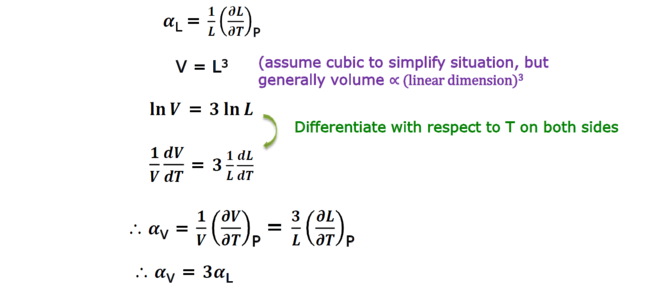## Want to keep learning?

This content is taken from the Hanyang University's online course, Thermodynamics in Energy Engineering. Join the course to learn more.
6.3

# Thermal expansion coefficient: derivation of relationship between linear expansion and volume expansion

$${ \alpha }_{ L }$$ VS $${ \alpha }_{ V }$$

In calculating entropy change for a solid under isothermal pressure change, we used thermal volume expansion coefficient ($${ \alpha }_{ V }$$). However, in most cases, not volume but linear expansion coefficients ($${ \alpha }_{ L }$$) are available. In the video, we used the relationship

$${ \alpha }_{ V }$$=3$${ \alpha }_{ L }$$

Here, let’s derive this relationship.